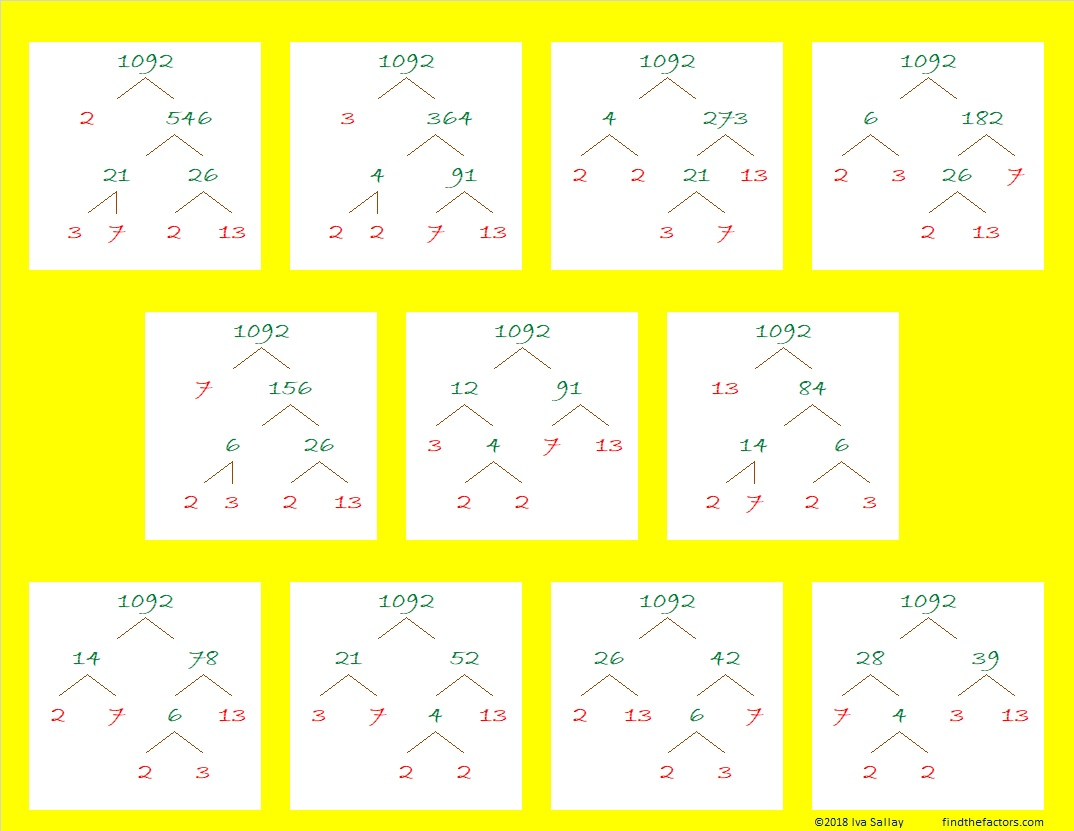1092 Predictable Factor Trees

A couple of years ago on Memorial Day weekend, we bought two peach trees from a local nursery. Those trees have grown bigger, and we will get some peaches this year. The smaller tree has lots of fruit growing on it and will need some attention because the branches will be too small to support the weight of all that fruit. The bigger tree has exactly one peach growing on it.

These factor trees for the number 1092 are a lot more predictable than those peach trees: No matter which of its factor pairs you use, you will always eventually get
2² × 3 × 7 × 13 = 1092.Here are some more facts about 1092:

• 1092 is a composite number.
• Prime factorization: 1092 = 2 × 2 × 3 × 7 × 13, which can be written 1092 = 2² × 3 × 7 × 13
• The exponents in the prime factorization are 2, 1, 1, and 1. Adding one to each and multiplying we get (2 + 1)(1 + 1)(1 + 1)(1 + 1) = 2 × 3 × 2 × 2 = 24. Therefore 1092 has exactly 24 factors.
• Factors of 1092: 1, 2, 3, 4, 6, 7, 12, 13, 14, 21, 26, 28, 39, 42, 52, 78, 84, 91, 156, 182, 273, 364, 546, 1092
• Factor pairs: 1092 = 1 × 1092, 2 × 546, 3 × 364, 4 × 273, 6 × 182, 7 × 156, 12 × 91, 13 × 84, 14 × 78, 21 × 52, 26 × 42, or 28 × 39
• Taking the factor pair with the largest square number factor, we get √1092 = (√4)(√273) = 2√273 ≈ 33.04542(12 × 13 × 14)/2 = 1092
Even though 1092 can claim that cool fact, it will actually make the next number a STAR!

1092 is the sum of the twelve prime numbers from 67 to 113:
67 + 71 + 73 + 79 + 83 + 89 + 97 + 101 + 103 + 107 + 109 + 113 = 1092

1092 has a lot of factors, but it is the hypotenuse of only one Pythagorean triple:
420-1008-1092 which is (5-12-13) times 84

Look how cool 1092 is in some other bases:

Not only does it use only 0’s and 1’s in base 2 but also in consecutive bases 3 and 4:
It’s 10001000100 in BASE 2 because 2¹º + 2⁶ + 2² = 1092,
1111110 in BASE 3 because 3⁶ + 3⁵ + 3⁴ + 3³ + 3² + 3¹ = 1092,
and 101010 in BASE 4 because  4⁵ + 4³ + 4¹ = 1092

I like the way it looks in consecutive bases 12 and 13:
It’s 770 in BASE 12, because 7(12² + 12) = 7(156) = 1092
and 660 in BASE 13 because 6(13² + 13) = 6(182) = 1092

And its repdigit 444 in BASE 16 because 4(16² + 16 + 1) = 1092

Some of these facts about 1092 were predictable and some were not, but I have enjoyed learning all of them and hope that you have too.